墨滴2021/04/24  阅读：79  主题：默认主题

# 拉格朗日插值法

### 插值法

#### 常用插值法

• 多项式插值： 为多项式函数
• 分段插值： 为分段多项式函数
• 三角插值： 为三角函数

#### 插值方法研究的问题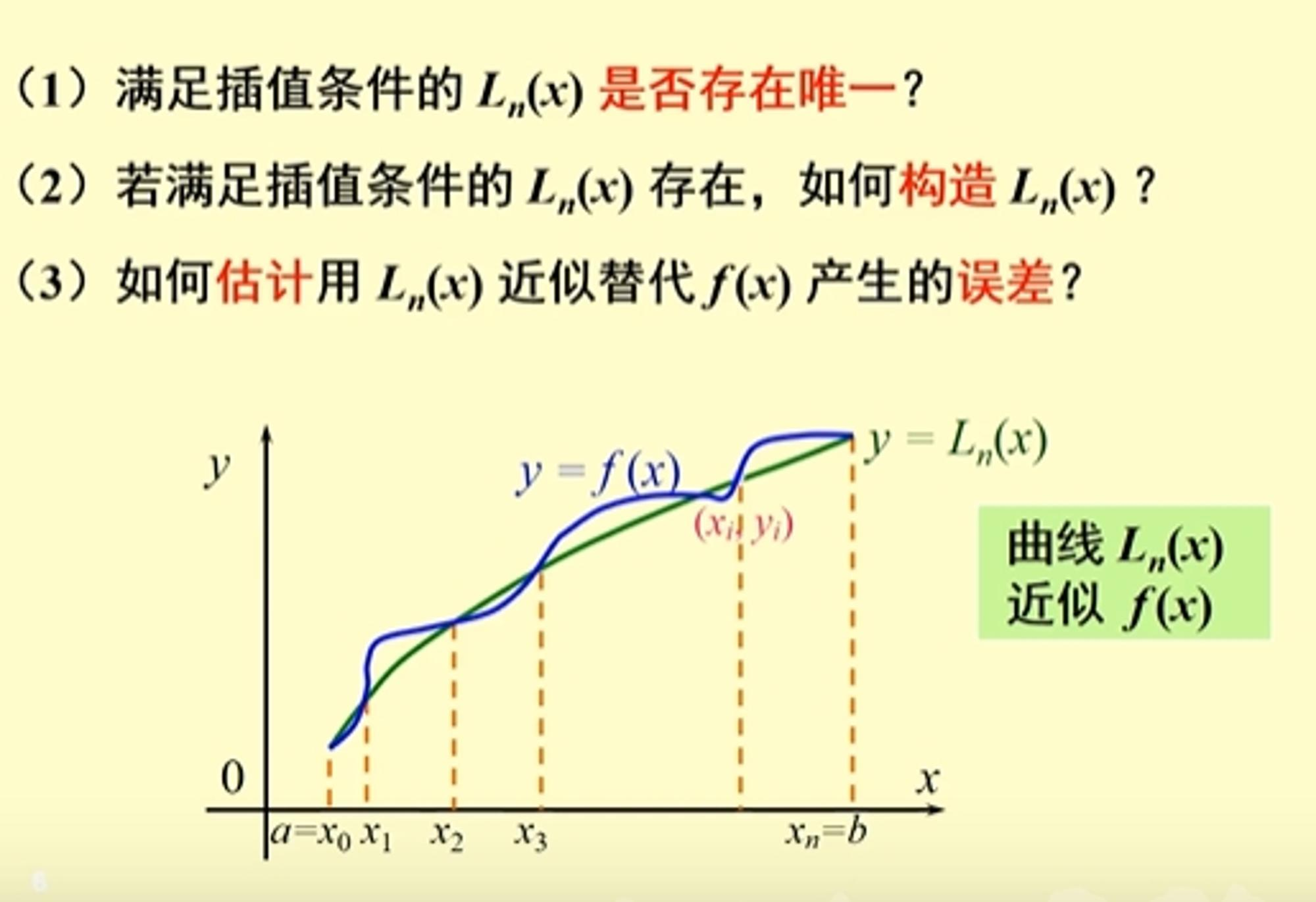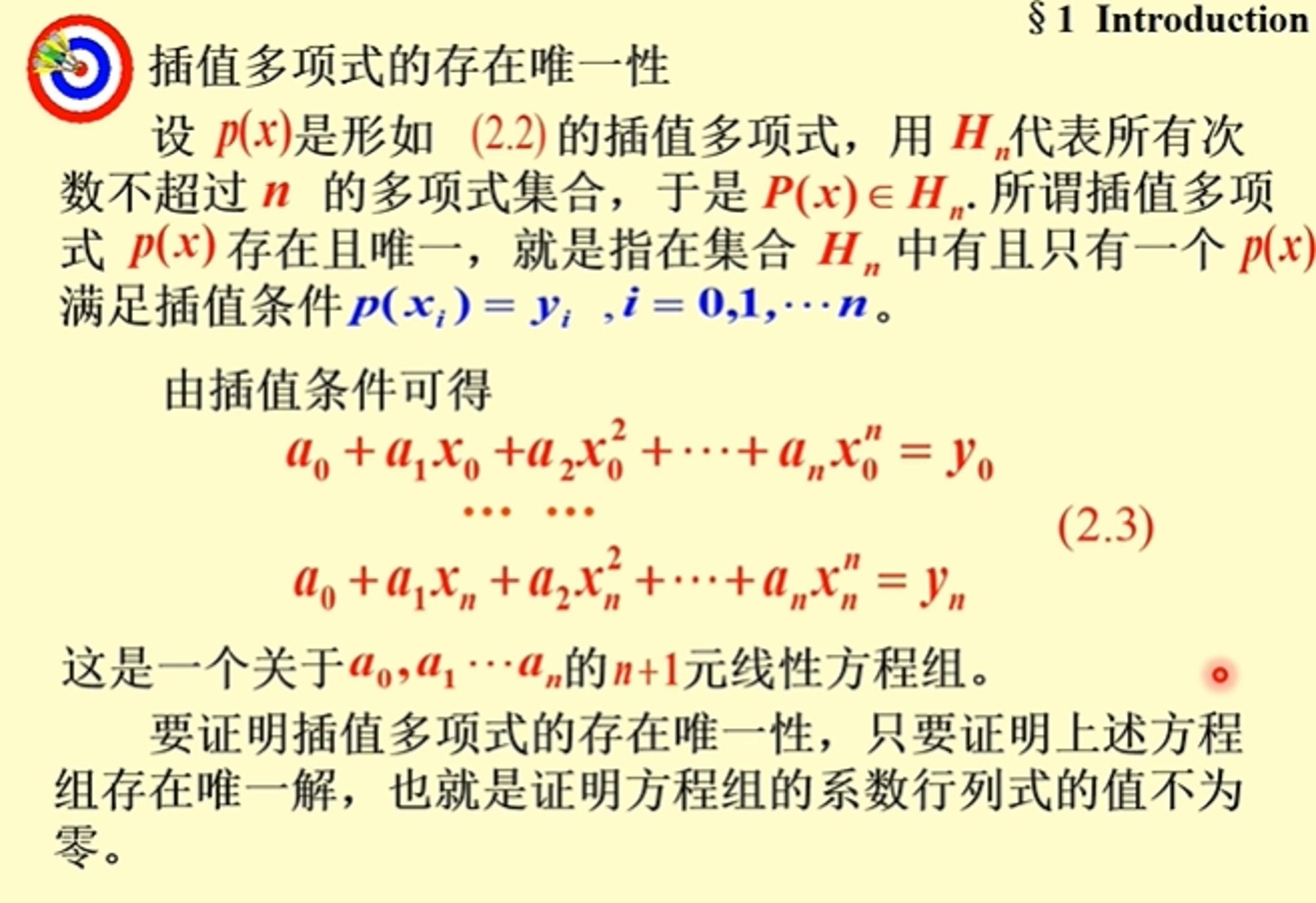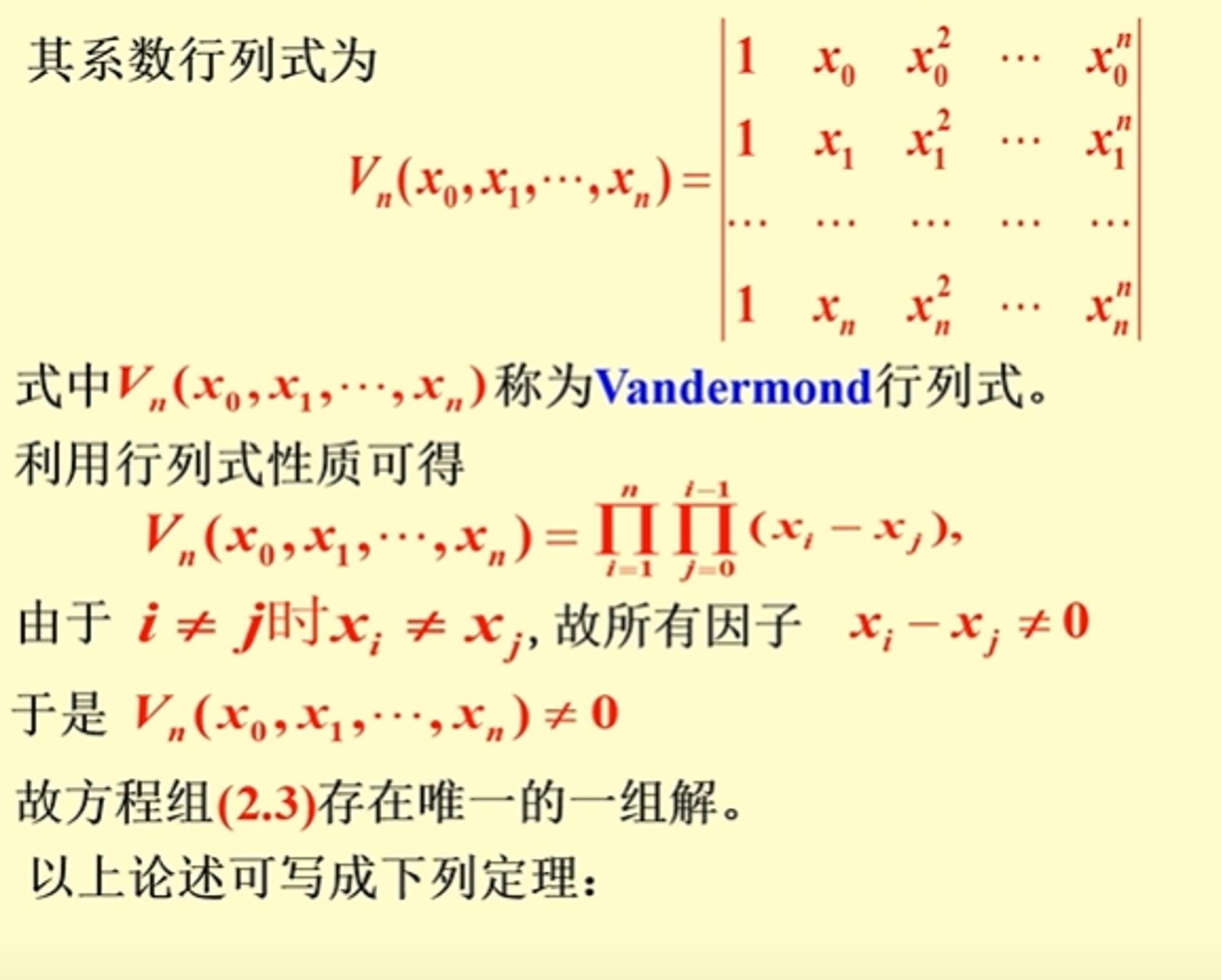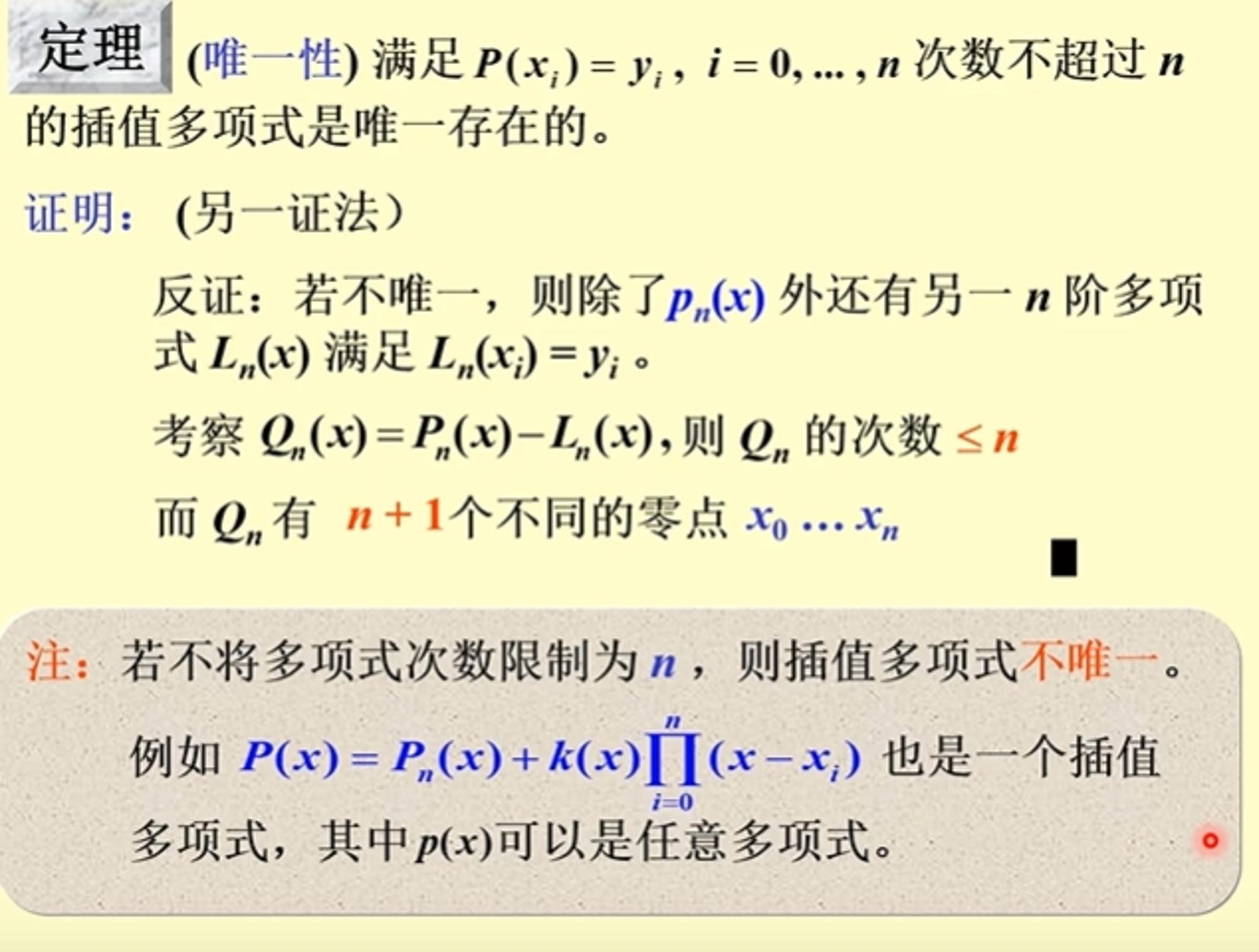拉格朗日多项式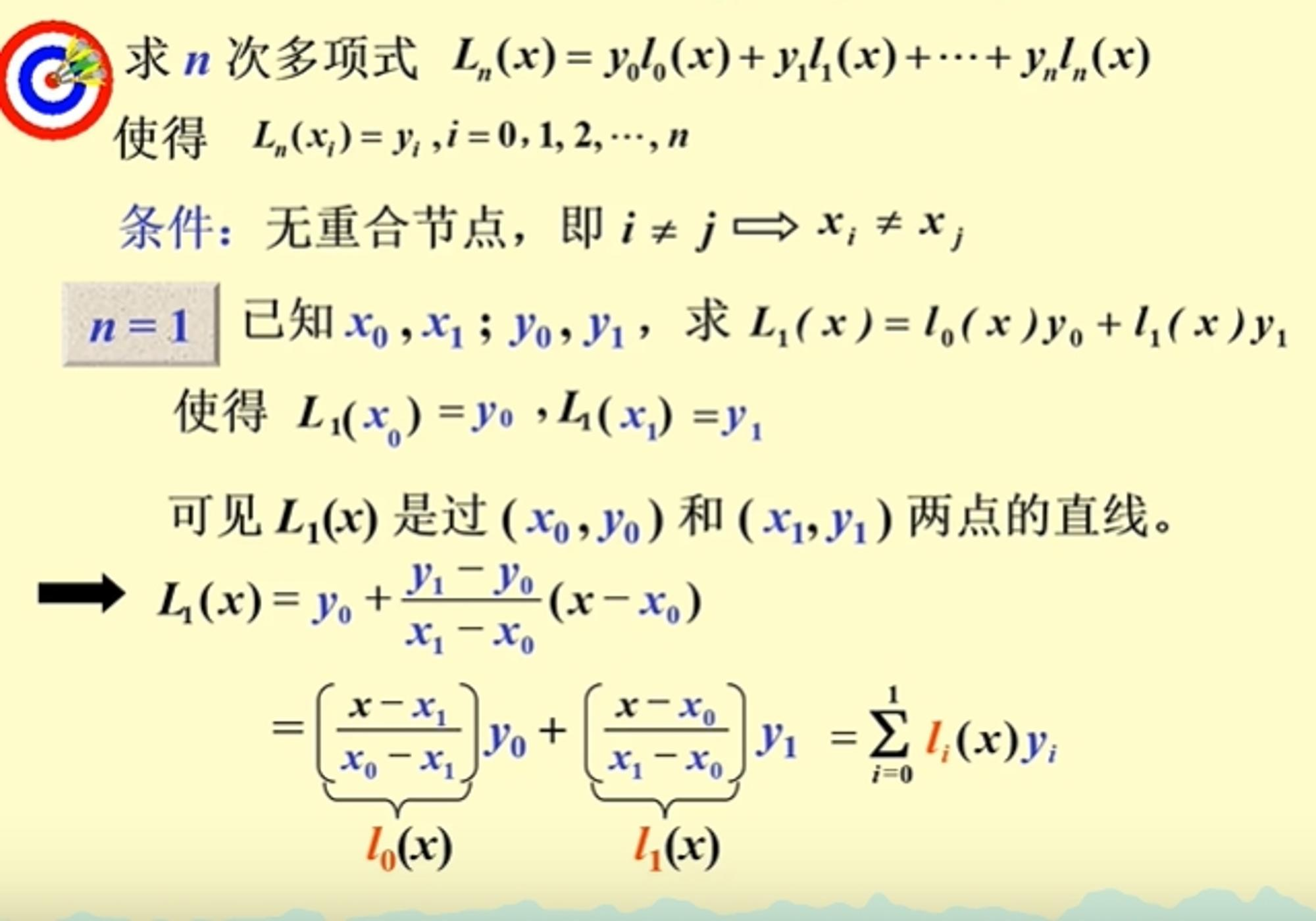函数称为拉氏基函数，满足条件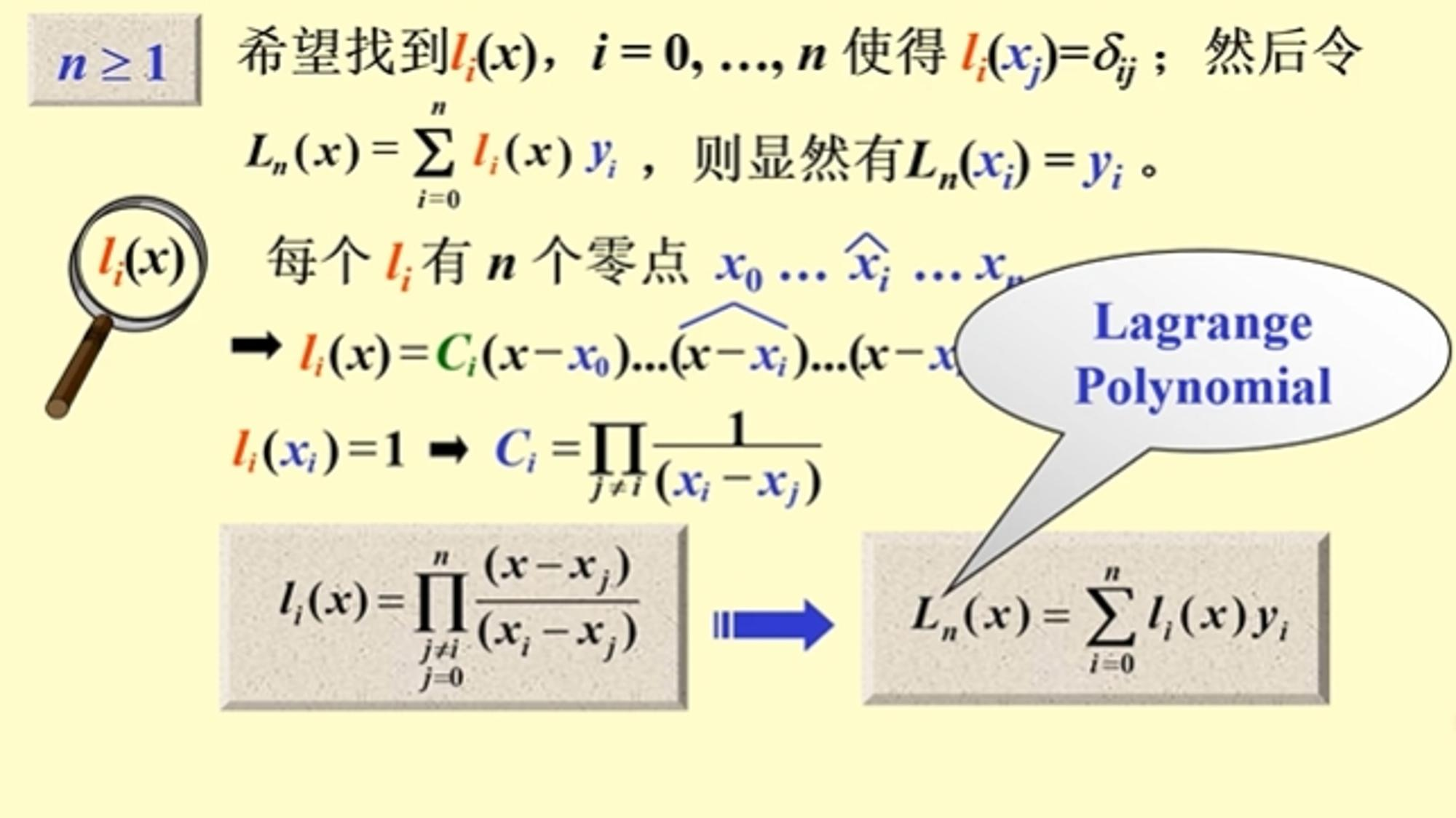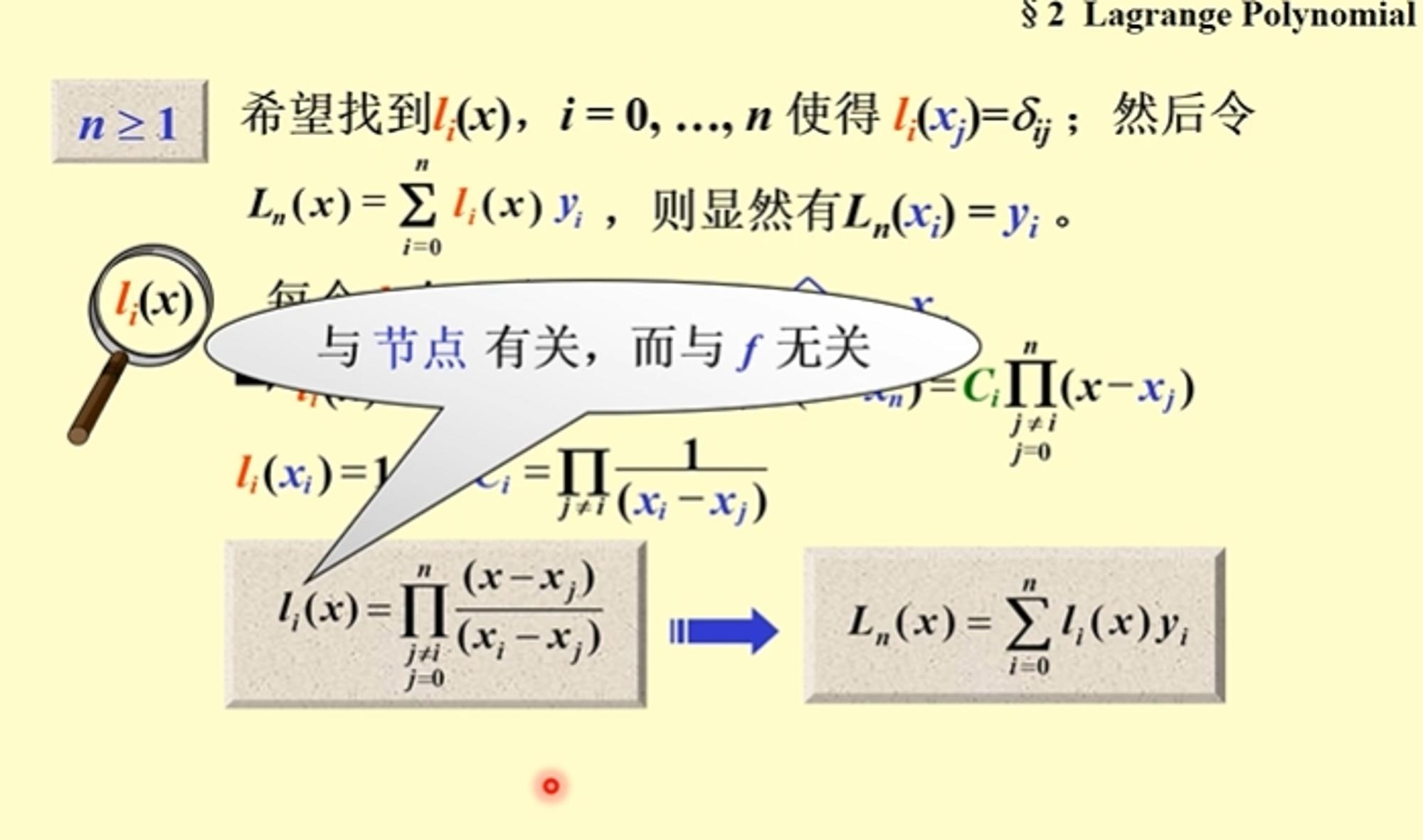注：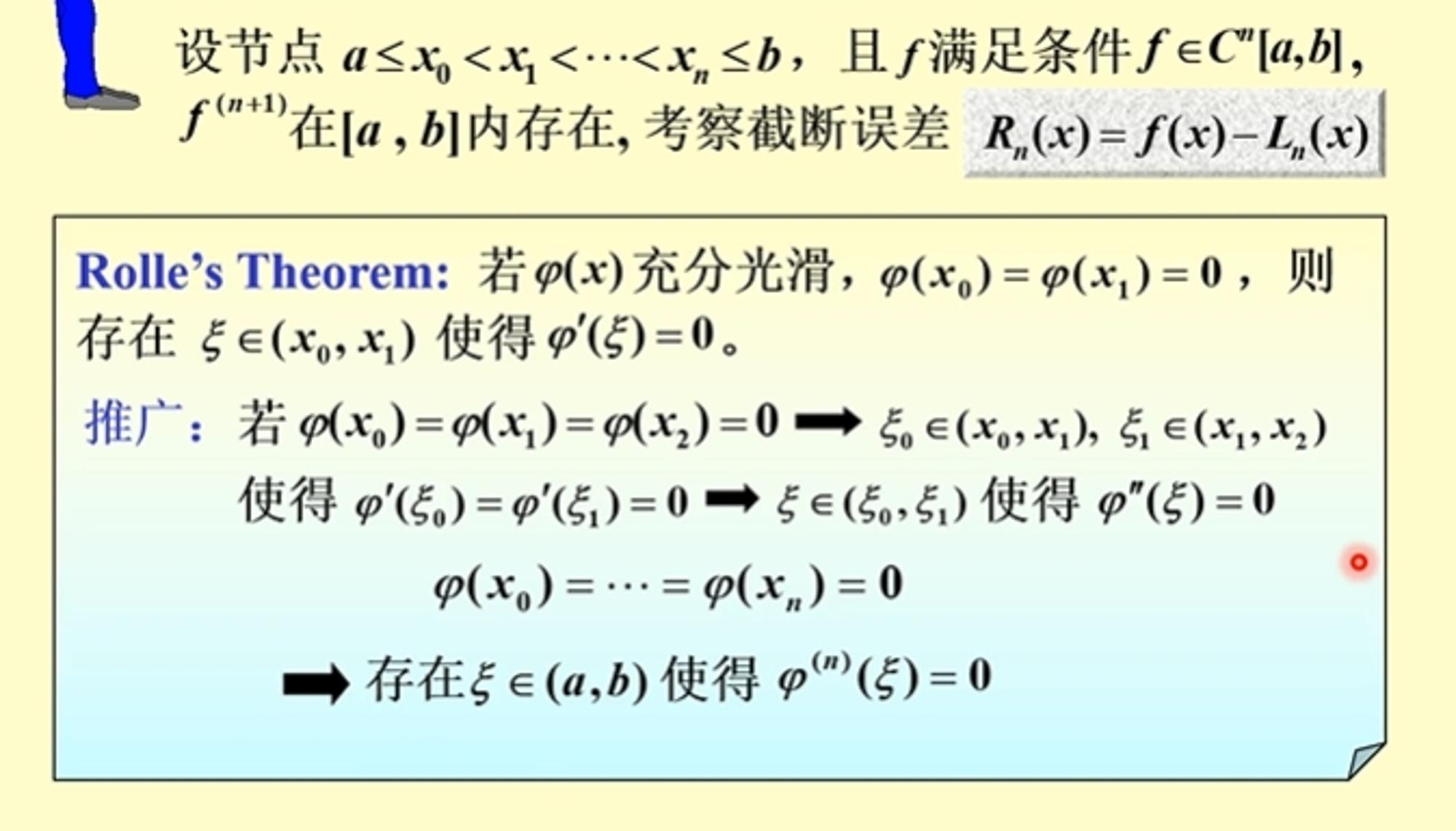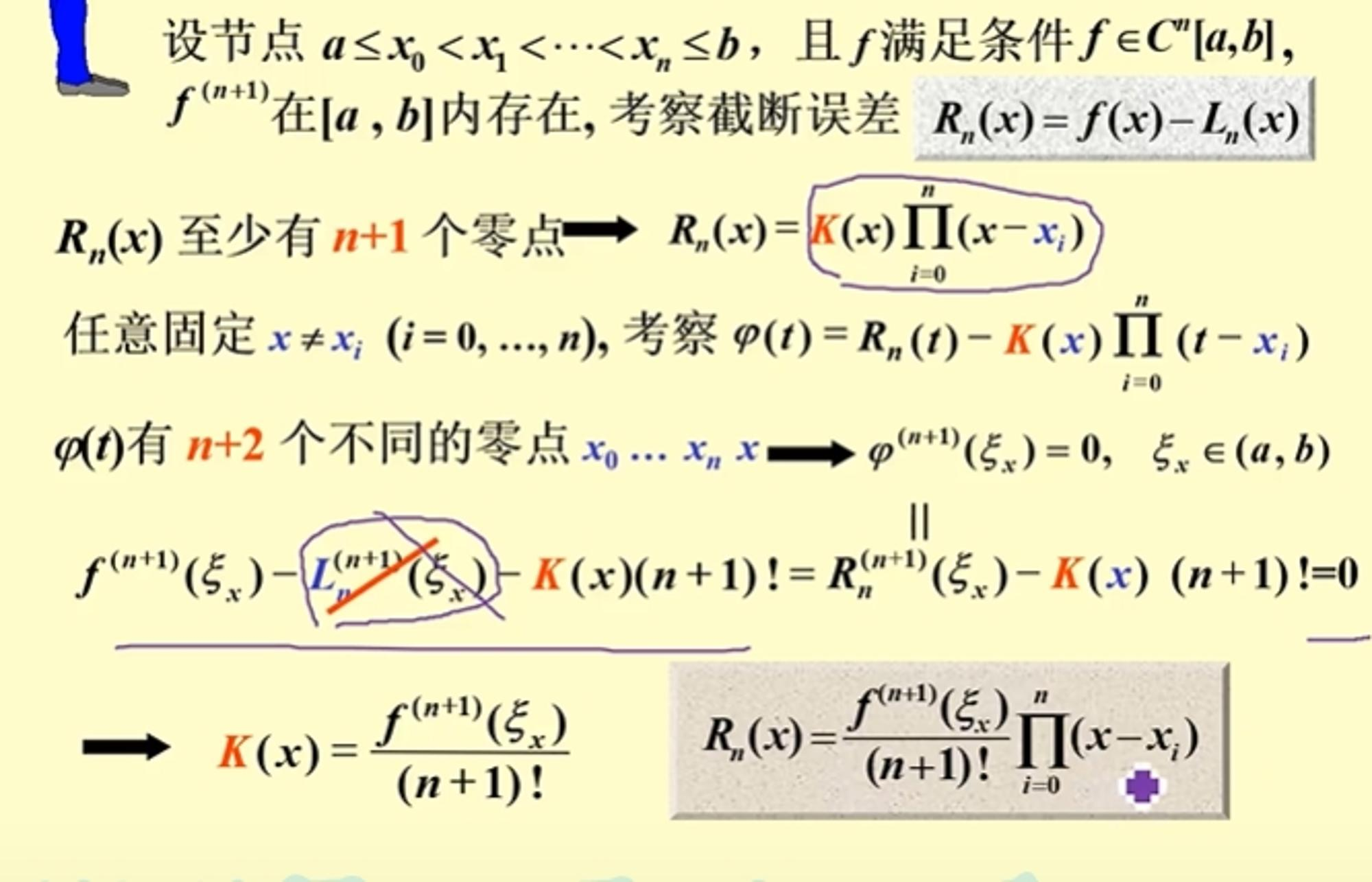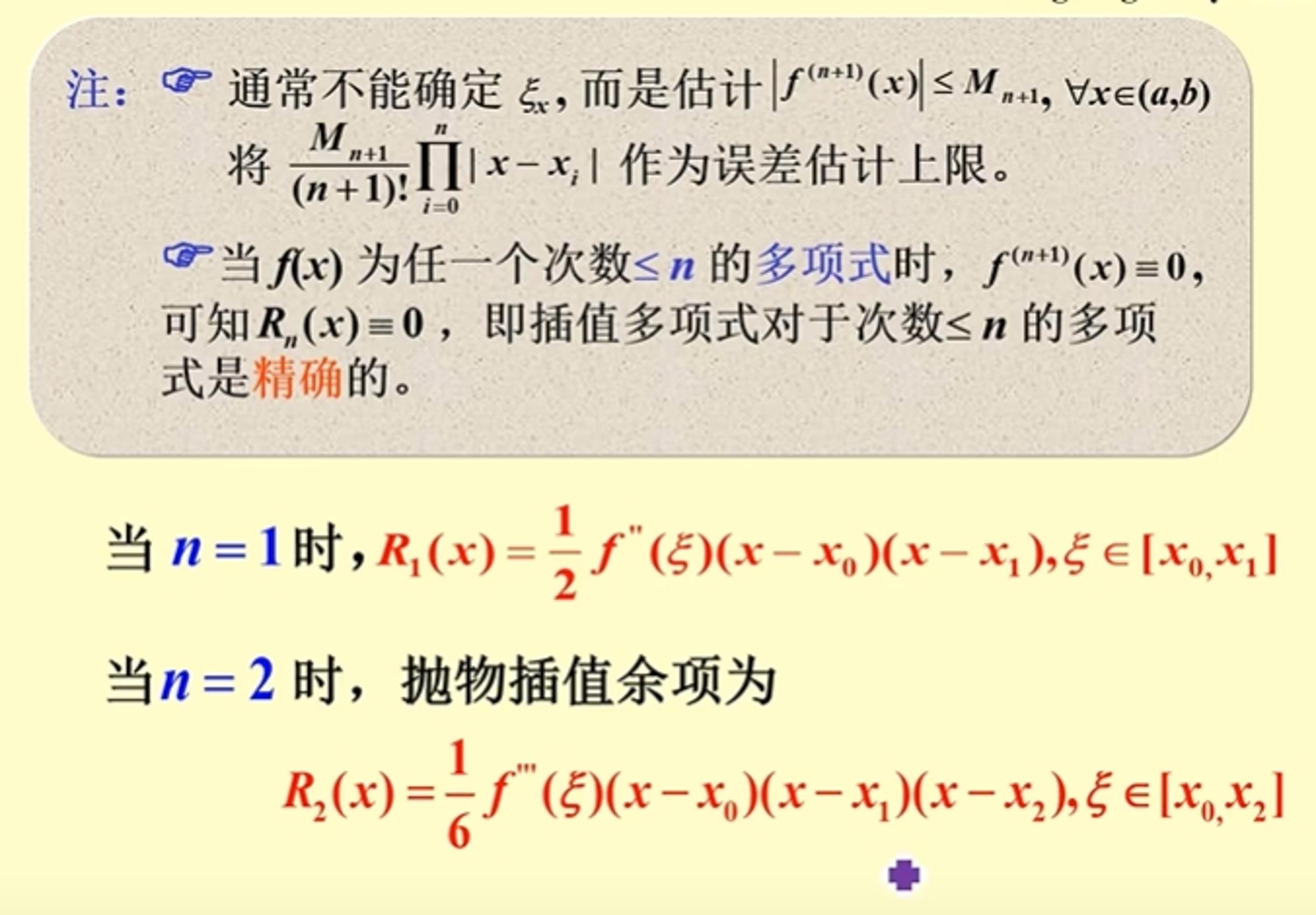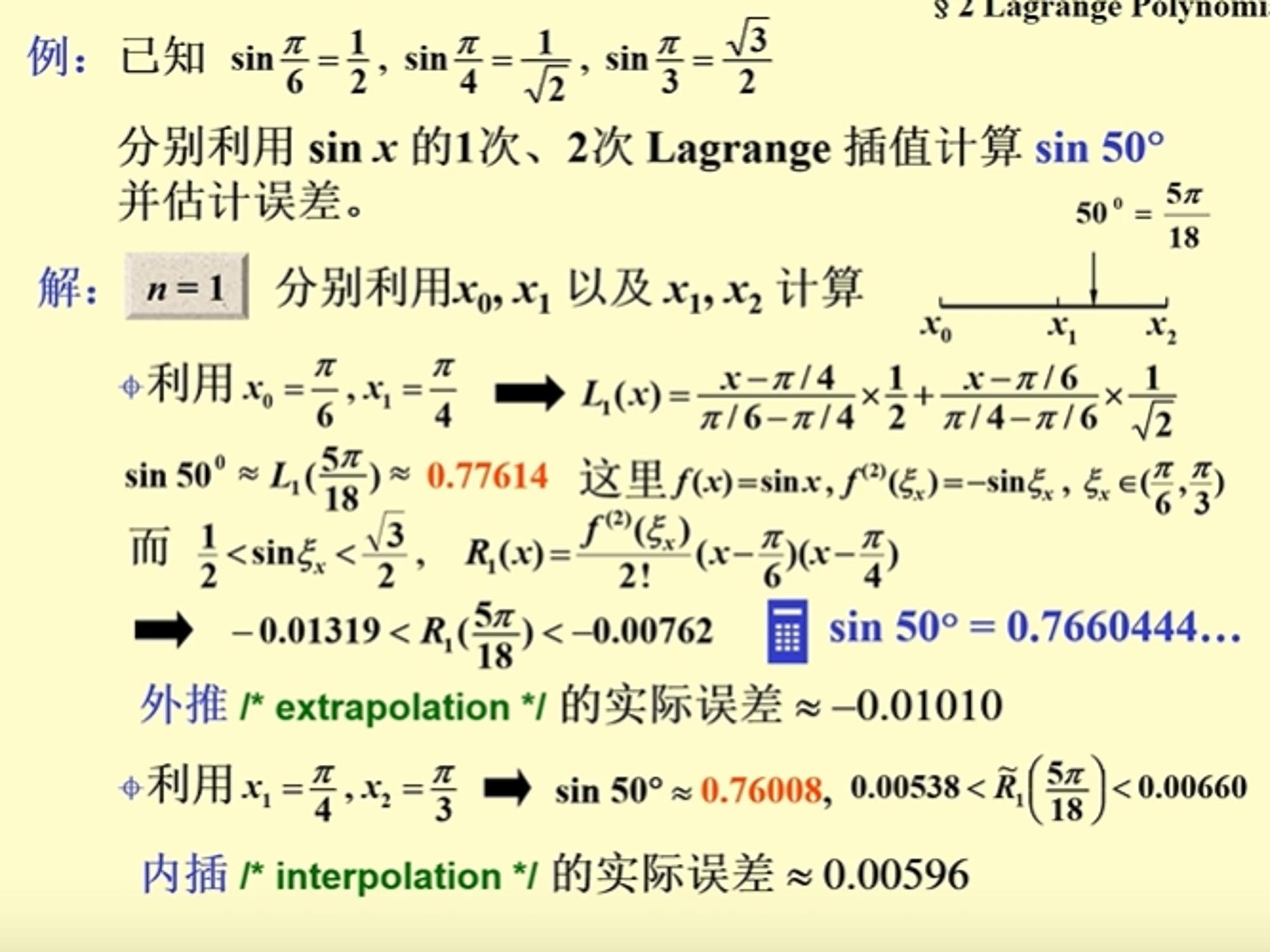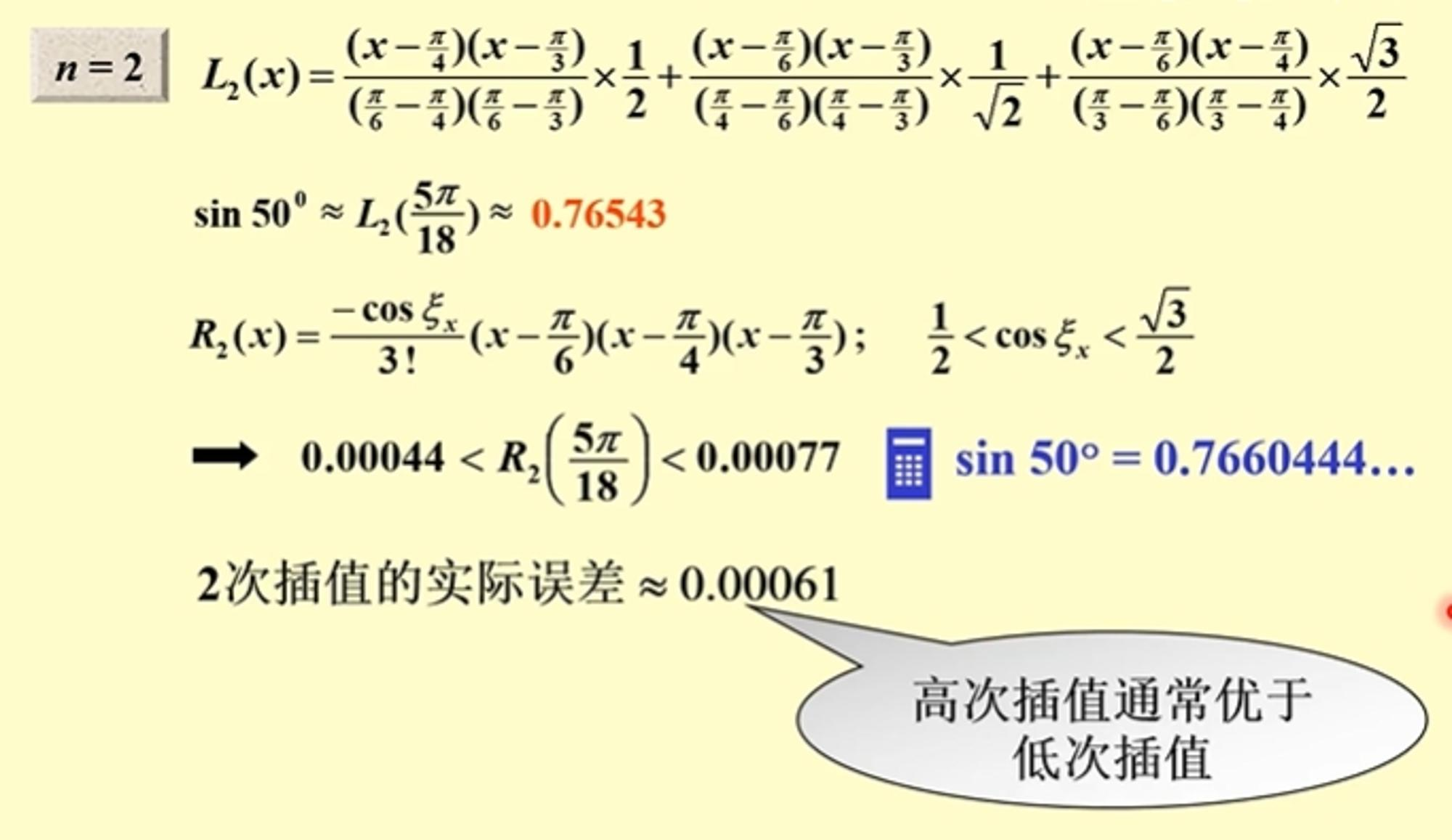#### matlab代码实现

function func = Lagrange_poly(X, y)    func = 0;    x = sym('x');    n = length(X);    for i = 1:n        f = 1;        for j = 1:n            if i~=j                f = f*(x-X(j))/(X(i)-X(j));            end        end        f = f*y(i);        func = func + f;    endend

X = [0, pi/6, pi/2, 5*pi/6, pi];Y = [0, 1/2 ,1, 1/2, 0];x = 0:pi/50:pi;f = Lagrange_poly(X, Y);y = subs(f, x);plot(x, sin(x));hold onplot(x, y);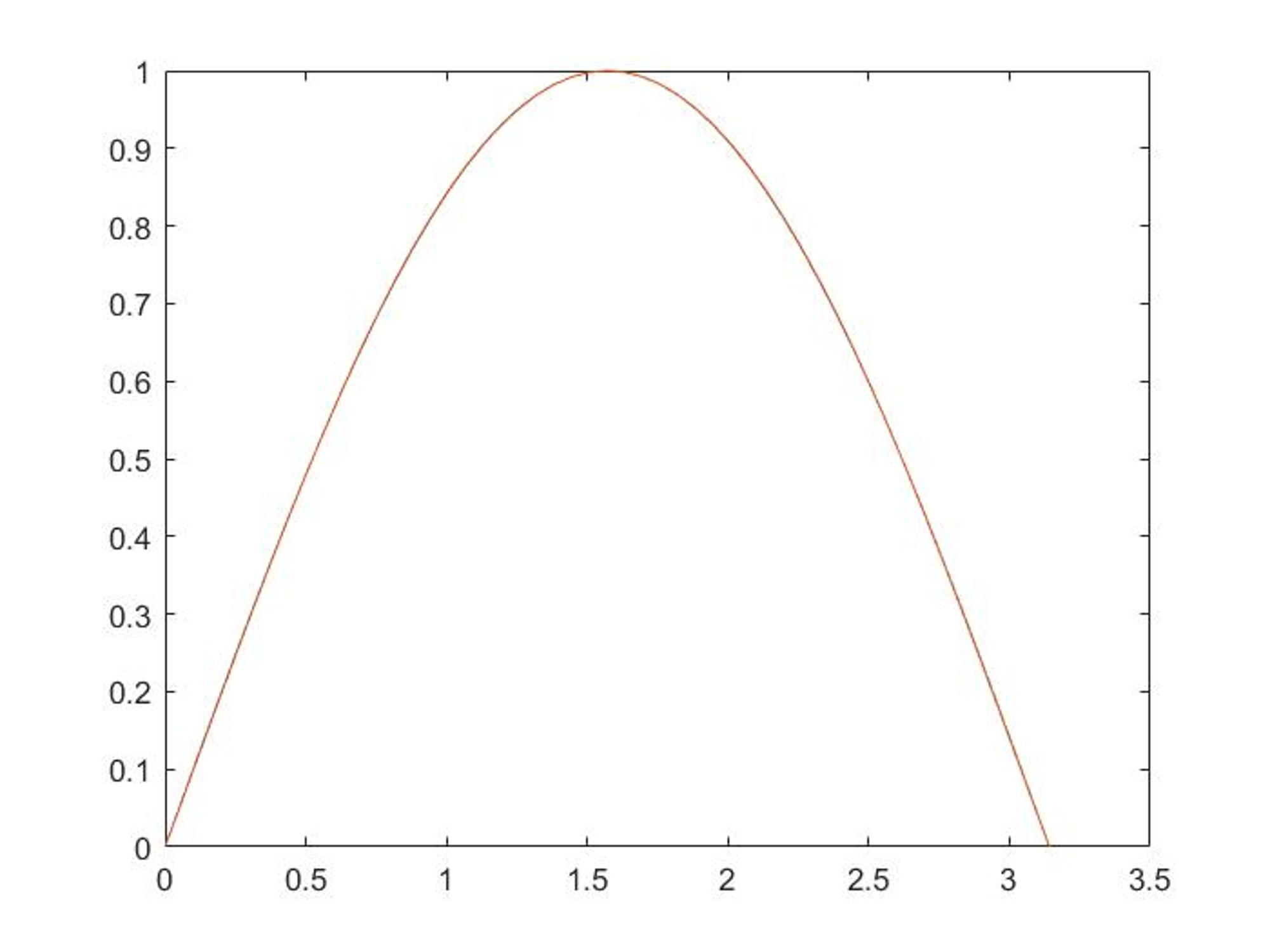得到的插值结果与原图基本一样。2021/04/24  阅读：79  主题：默认主题